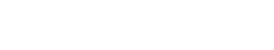# Semiparametric Models: Simple Definition and Examples

A semiparametric model is a regression model with both a finite- and an infinite-dimensional component.

A finite-dimensional component is spanned by some list of vectors (a vector is an object that has both magnitude and direction). The two-dimensional and three-dimensional spaces we deal with in everyday geometry are examples of finite-dimensional spaces, but so is a hypothetical 4568-dimensional space. Infinite dimensional spaces are spaces that have an infinite, and possibly ill-defined, number of dimensions and possibilities. They aren’t spanned by any finite list of vectors.

In contrast to parametric models, which are well-defined in the finite-dimensional space, and non-parametric models, where the parameters can all span an infinite space, a semiparametric model has a component that is finite-dimensional (i.e. it’s easy to research and understand), and another that is infinite-dimensional (i.e. beyond the range of ordinary statistical methods).

In research and statistics projects involving semi-parametric models the emphasis is almost always on the parametric component of the model. That’s because this is the part which lends itself well to research.

## Why Use Semiparametric Models?

Too often parametric models, while being easy to understand and easy to work with, fail to give a fair representation of what is happening in the real world. Non-parametric models may be better representations but do not lend themselves well to analysis. A semiparametric model allows you to have the best of both worlds: a model that is understandable and can be manipulated while still offering a fair representation of the messiness that is involved in real life.

## Examples of Semiparametric Models

One example of a semi-parametric model is the Cox Proportional Hazards Model. This model is very useful in studies of the time remaining before an end or failure; it’s used when doing research on the time remaining before a patient dies, or before a light bulb burns out. It’s defined as:Here x is what we call the covariate vector, and our unknown parameters are Β and λ0 (u). Β is finite-dimensional and is often the subject of research; λ0 (u), on the other hand, is a unknown, non-negative function of time, and the space of λ0 (u) possibilities is infinite. We call it a ‘nuisance parameter‘; a parameter that can’t be entirely omitted in a rigorous treatment of a problem but is not of immediate interest– typically because it is difficult or impossible to study.

Gaussian mixture models are semi-parametric. Parametric implies that the model comes from a known distribution (which is in this case, a set of normal distributions). It’s semi-parametric because more components, possibly from unknown distributions, can be added to the model.

## References

Vector Algebra

CITE THIS AS:
Stephanie Glen. "Semiparametric Models: Simple Definition and Examples" From StatisticsHowTo.com: Elementary Statistics for the rest of us! https://www.statisticshowto.com/semiparametric/
---------------------------------------------------------------------------Need help with a homework or test question? With Chegg Study, you can get step-by-step solutions to your questions from an expert in the field. Your first 30 minutes with a Chegg tutor is free!